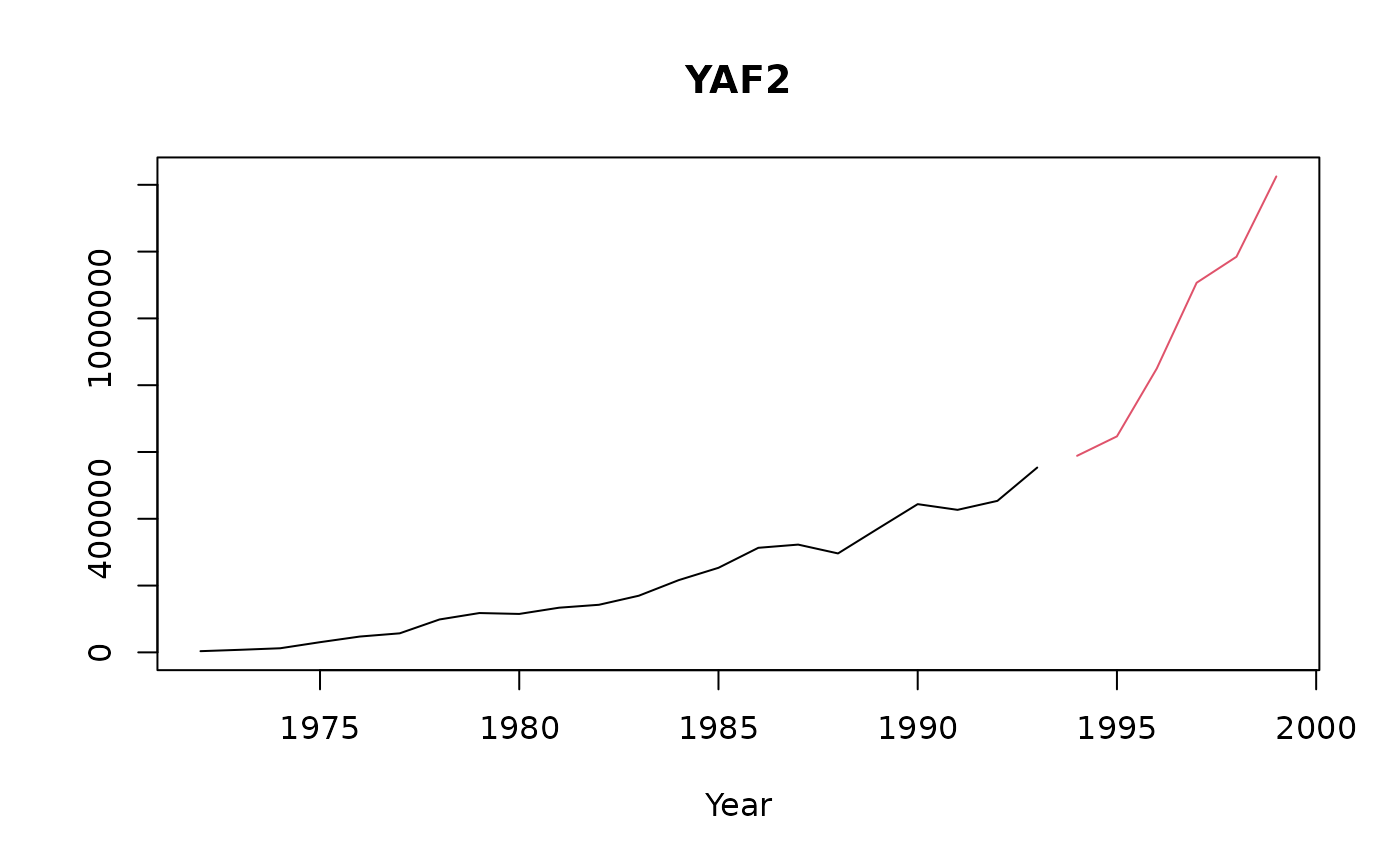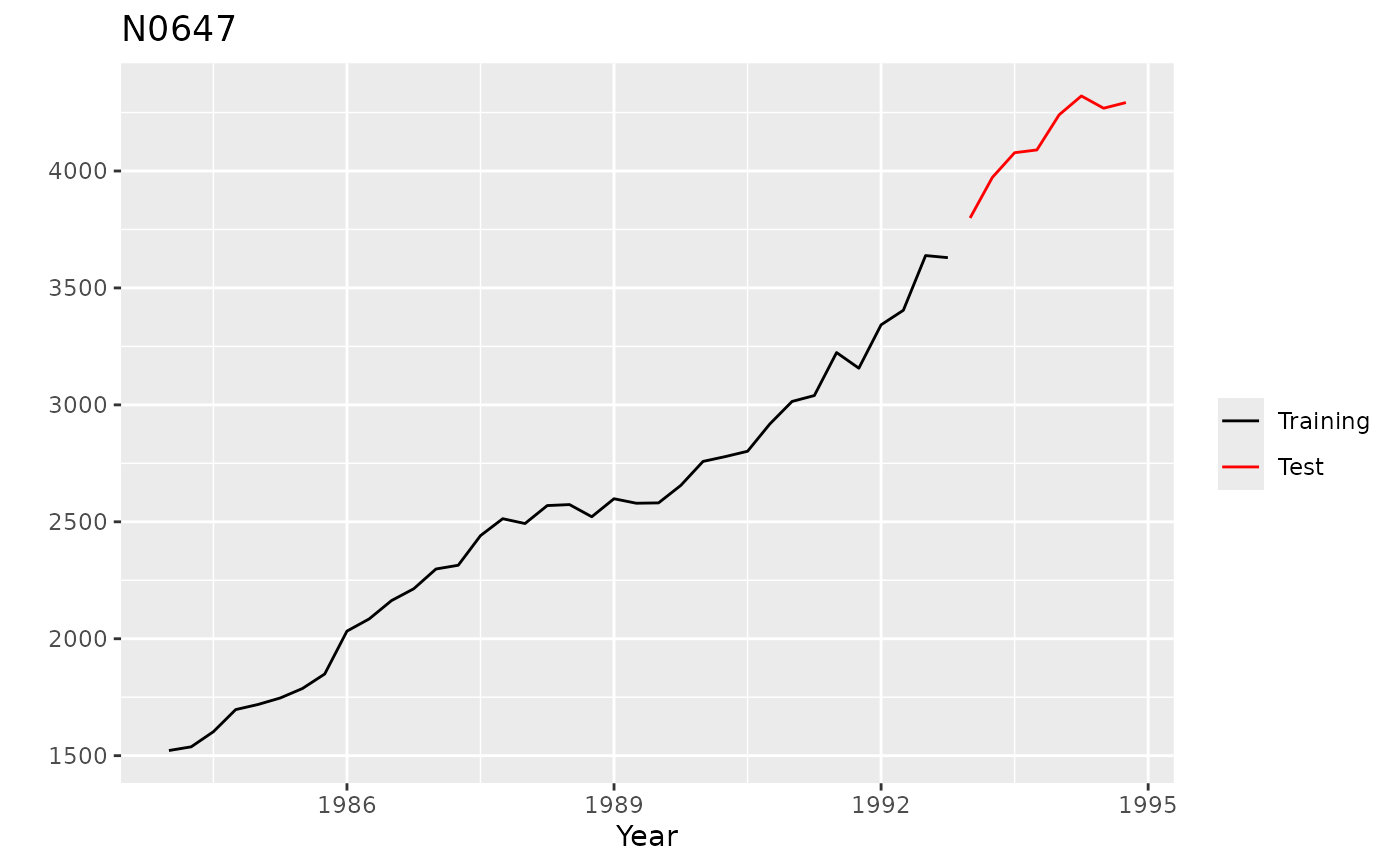Functions to plot a time series from the M competition data sets, showing both the training and test sections of the series.

# S3 method for Mdata
plot(x, xlim = c(tsp(x$x), tsp(x$xx)),
ylim = range(x$x, x$xx), main = x$sn, xlab, ylab = "", ...) # S3 method for Mdata autoplot(object, ...) Arguments x, object A series of M-competition data Limits on x-axis Limits on y-axis Main title Label on x-axis Label on y-axis Other plotting arguments passed to plot. Ignored for autoplot. Value autoplot.Mdata returns a ggplot2 object, while plot.Mdata returns nothing. Both functions produce a time series plot of the selected series. See also Examples library(ggplot2) plot(M1[])autoplot(M1$YAF3)autoplot(M3[["N0647"]])